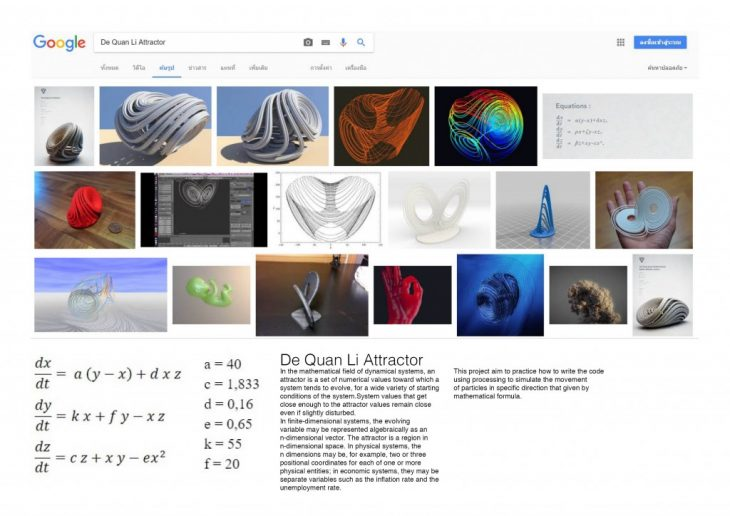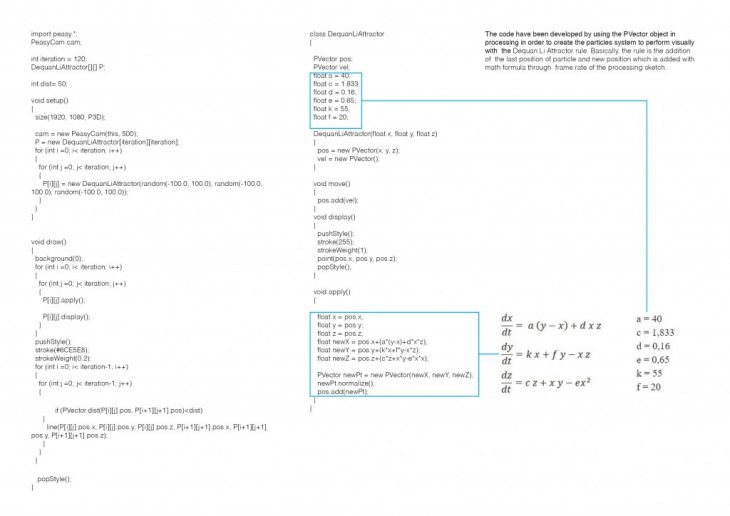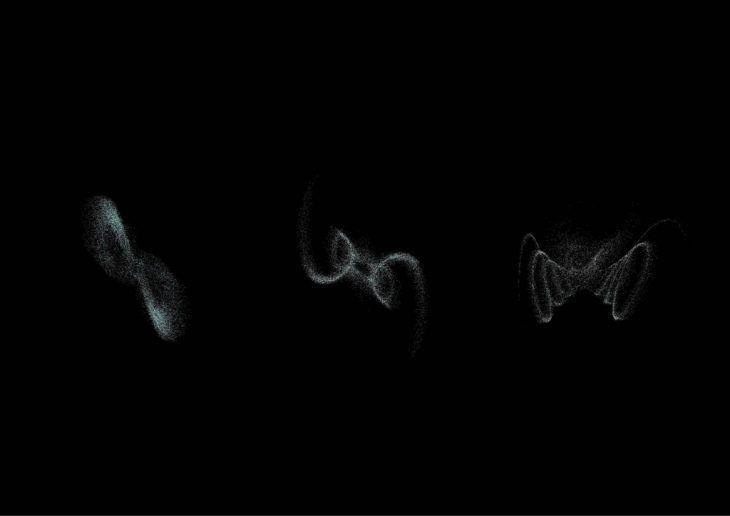De Quan Li Attractor
In the mathematical field of dynamical systems, an attractor is a set of numerical values toward which a system tends to evolve, for a wide variety of starting conditions of the system.System values that get close enough to the attractor values remain close even if slightly disturbed.
In finite-dimensional systems, the evolving variable may be represented algebraically as an n-dimensional vector. The attractor is a region in n-dimensional space. In physical systems, the n dimensions may be, for example, two or three positional coordinates for each of one or more physical entities; in economic systems, they may be separate variables such as the inflation rate and the unemployment rate.

This project aim to practice how to write the code using processing to simulate the movement of particles in specific direction that given by mathematical formula.The code have been developed by using the PVector object in processing in order to create the particles system to perform visually with the Dequan Li Attractor rule. Basically, the rule is the addition of the last position of particle and new position which is added with math formula through frame rate of the processing sketch.De Quan Li Attractor is a project of IaaC, Institute for Advanced Architecture of Catalonia, developed at Master in Advanced Architecture in 2015/2017 by: Introductory to Processing 2016 : Assignment 3 Student: Inthat Ueasak-aree Faculty: Angelos Chronis